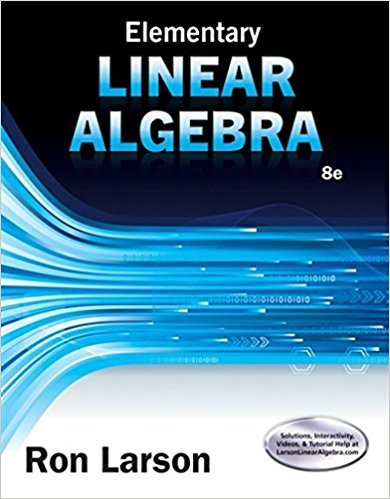×
×

# Solutions for Chapter 5.4: Mathematical Models and Least Squares Analysis## Full solutions for Elementary Linear Algebra | 8th Edition

ISBN: 9781305658004Solutions for Chapter 5.4: Mathematical Models and Least Squares Analysis

Solutions for Chapter 5.4
4 5 0 240 Reviews
10
0
##### ISBN: 9781305658004

Elementary Linear Algebra was written by and is associated to the ISBN: 9781305658004. This textbook survival guide was created for the textbook: Elementary Linear Algebra, edition: 8. Chapter 5.4: Mathematical Models and Least Squares Analysis includes 96 full step-by-step solutions. Since 96 problems in chapter 5.4: Mathematical Models and Least Squares Analysis have been answered, more than 44867 students have viewed full step-by-step solutions from this chapter. This expansive textbook survival guide covers the following chapters and their solutions.

Key Math Terms and definitions covered in this textbook
• Back substitution.

Upper triangular systems are solved in reverse order Xn to Xl.

• Cholesky factorization

A = CTC = (L.J]))(L.J]))T for positive definite A.

• Cramer's Rule for Ax = b.

B j has b replacing column j of A; x j = det B j I det A

• Cyclic shift

S. Permutation with S21 = 1, S32 = 1, ... , finally SIn = 1. Its eigenvalues are the nth roots e2lrik/n of 1; eigenvectors are columns of the Fourier matrix F.

• Determinant IAI = det(A).

Defined by det I = 1, sign reversal for row exchange, and linearity in each row. Then IAI = 0 when A is singular. Also IABI = IAIIBI and

• Dot product = Inner product x T y = XI Y 1 + ... + Xn Yn.

Complex dot product is x T Y . Perpendicular vectors have x T y = O. (AB)ij = (row i of A)T(column j of B).

• Elimination.

A sequence of row operations that reduces A to an upper triangular U or to the reduced form R = rref(A). Then A = LU with multipliers eO in L, or P A = L U with row exchanges in P, or E A = R with an invertible E.

• Fast Fourier Transform (FFT).

A factorization of the Fourier matrix Fn into e = log2 n matrices Si times a permutation. Each Si needs only nl2 multiplications, so Fnx and Fn-1c can be computed with ne/2 multiplications. Revolutionary.

• Graph G.

Set of n nodes connected pairwise by m edges. A complete graph has all n(n - 1)/2 edges between nodes. A tree has only n - 1 edges and no closed loops.

• Hilbert matrix hilb(n).

Entries HU = 1/(i + j -1) = Jd X i- 1 xj-1dx. Positive definite but extremely small Amin and large condition number: H is ill-conditioned.

• Hypercube matrix pl.

Row n + 1 counts corners, edges, faces, ... of a cube in Rn.

• Least squares solution X.

The vector x that minimizes the error lie 112 solves AT Ax = ATb. Then e = b - Ax is orthogonal to all columns of A.

• Minimal polynomial of A.

The lowest degree polynomial with meA) = zero matrix. This is peA) = det(A - AI) if no eigenvalues are repeated; always meA) divides peA).

• Network.

A directed graph that has constants Cl, ... , Cm associated with the edges.

• Normal matrix.

If N NT = NT N, then N has orthonormal (complex) eigenvectors.

• Orthogonal matrix Q.

Square matrix with orthonormal columns, so QT = Q-l. Preserves length and angles, IIQxll = IIxll and (QX)T(Qy) = xTy. AlllAI = 1, with orthogonal eigenvectors. Examples: Rotation, reflection, permutation.

• Permutation matrix P.

There are n! orders of 1, ... , n. The n! P 's have the rows of I in those orders. P A puts the rows of A in the same order. P is even or odd (det P = 1 or -1) based on the number of row exchanges to reach I.

• Positive definite matrix A.

Symmetric matrix with positive eigenvalues and positive pivots. Definition: x T Ax > 0 unless x = O. Then A = LDLT with diag(D» O.

• Schur complement S, D - C A -} B.

Appears in block elimination on [~ g ].

• Skew-symmetric matrix K.

The transpose is -K, since Kij = -Kji. Eigenvalues are pure imaginary, eigenvectors are orthogonal, eKt is an orthogonal matrix.

×